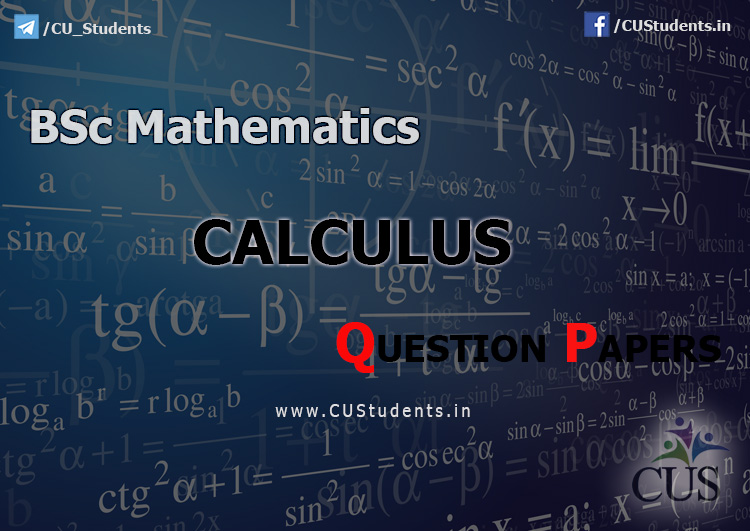# BSc Mathematics - Calculus - Previous Question PapersBSc Mathematics Calculus 2017
BSc Mathematics Calculus 2016
BSc Mathematics Calculus 2015
BSc Mathematics Calculus 2014
BSc Mathematics Calculus 2013
BSc Mathematics Calculus 2012
BSc Mathematics Calculus 2011
BSc Mathematics Calculus 2010

BSc Mathematics Calculus 2016

BSc Mathematics Calculus 2015Balas

I cannot open bsc mathematics 6th semesterBalas

I can't open 6th sem orevprev yr qp ..pls hekpBalas

I want bsc mathematics 6th semester question papper of number theory and linear algebraBalas